Question

A car at speed v takes a turn of radius R on a banked road of angle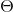. What is the angle that the road must be banked ti bit require the driver to turn the steering wheel? For circular motion, the centripetal acceleration is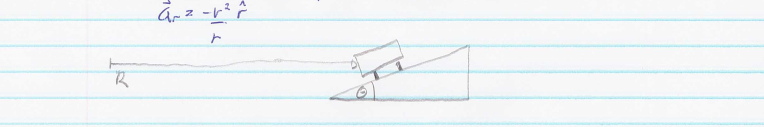Now the road has a coefficient of friction of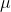with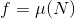. What is the maximum velocity that the driver can take the turn?

Free body diagram of the car in absence and in presence of frictional force is shown in the figure......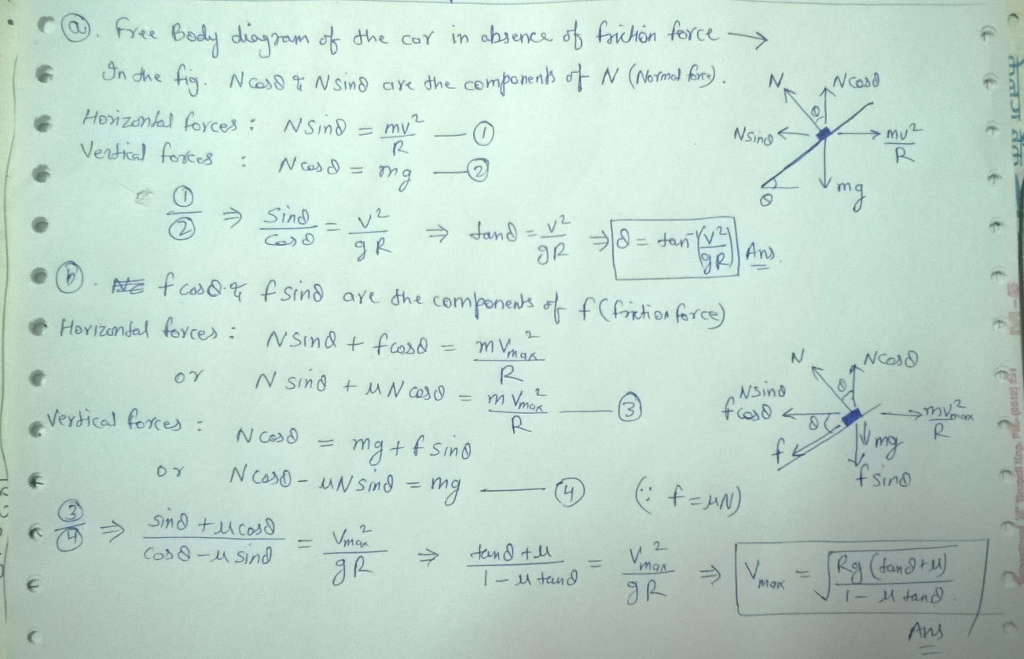#### Earn Coins

Coins can be redeemed for fabulous gifts.

Similar Homework Help Questions
• ### A car of mass M= 1300 kg traveling at 65.0 km/hour enters a banked turn covered with ice. The road is banked at an ang...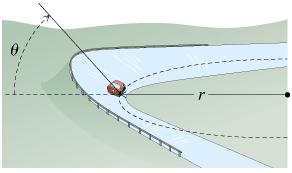A car of mass M= 1300 kg traveling at 65.0 km/hour enters a banked turn covered with ice. The road is banked at an angle , and there is no friction between the road and the car's tires. (Intro 1 figure) . Use g= 9.80 m/s^2 throughout this problem. What is the radius (in meters) of the turn if = 20.0 (assuming the car continues in uniform circular motion around the turn)?

• ### A car of mass M = 1500 kg traveling at 65.0 km/hour enters a banked turn...

A car of mass M = 1500 kg traveling at 65.0 km/hour enters a banked turn covered with ice. The road is banked at an angle ∘, and there is no friction between the road and the car's tires as shown in (Figure 1). Use g = 9.80 m/s2 throughout this problem. What is the radius r of the turn if θ = 20.0 ∘ (assuming the car continues in uniform circular motion around the turn)?

• ### 1) A road is banked at 10 degrees at a circular turn of radius 100m. a....

1) A road is banked at 10 degrees at a circular turn of radius 100m. a. What is the exact speed required such that the car stays on the road when it is icy and without friction? b. What minimum coefficeient of static friction is needed if the car travels at exactly one half the speed you found in a.

• ### A car rounds a curve that is banked inward. The radius of curvature of the road...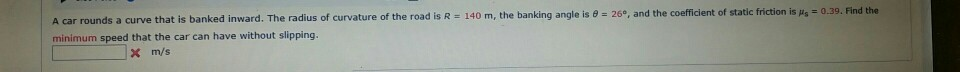A car rounds a curve that is banked inward. The radius of curvature of the road is R = 140 m, the banking angle is θ = 26°, and the coefficient of static friction is μs = 0.39. Find the minimum speed that the car can have without slipping. A car rounds a curve that is banked inward. The radius of curvature of the road is R 140 m, the banking angle is 26e, and the coefficient of static minimum...

• ### 2. A car travels on a rough banked curved road of radius 200 m with velocity...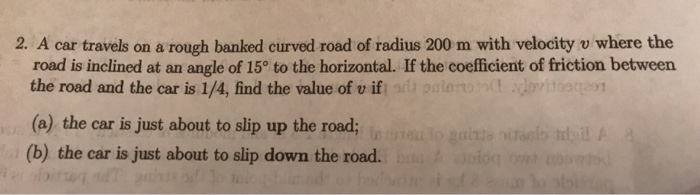2. A car travels on a rough banked curved road of radius 200 m with velocity v where the road is inclined at an angle of 15° to the horizontal. If the coefficient of friction betweern the road and the car is 1/4, find the value of v if (a) the car is just about to slip up the road; (b) the car is just about to slip down the road.

• ### We are building a road, and at one place we need to make a turn with...

We are building a road, and at one place we need to make a turn with a radius of 30m.  The coefficient of static friction between rubber and the material we are using is 0.6. Instead of keeping the road flat, let's bank the turn a little bit, giving the road an angle of 20﻿∘ above the horizontal. With the banked turn, what is the maximum speed a car could have and still make it around the turn without sliding?

• ### 7. A highway curve with a radius of R metres is banked so that cars moving at v m/s around the curve do not have to rely on friction when taking the turn. IWPS 7. No.4] 7.1 Show (from first principle...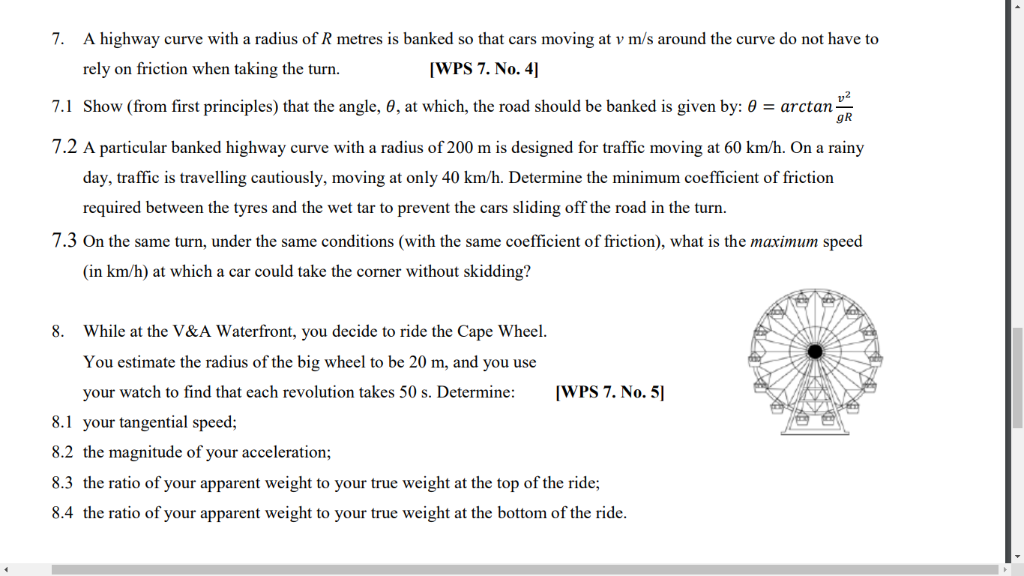7. A highway curve with a radius of R metres is banked so that cars moving at v m/s around the curve do not have to rely on friction when taking the turn. IWPS 7. No.4] 7.1 Show (from first principles) that the angle, 6, at which, the road should be banked is given by: 0 arctan 7.2 A particular banked highway curve with a radius of 200 m is designed for traffic moving at 60 km/h. On a rainy...

• ### Banked curves are designed so that the radial component of the normal force on the car rounding

Banked curves are designed so that the radial component of the normal force on the car rounding the curve provides the centripetal force required to execute uniform clrcular motion and safely negotlate the curve. A car rounds a banked curve with banking angle θ-27.1° and radius of curvature 157 m. (a) It the coefficient of static friction between the car's tires and the road is -0.316, what is the range ot speeds for which the car can safely negotiate the turn...

• ### A car rounds a curve that is banked inward. The radius of curvature of the road...

A car rounds a curve that is banked inward. The radius of curvature of the road is R = 152 m, the banking angle is θ = 32°, and the coefficient of static friction is μs = 0.23. Find the minimum speed that the car can have without slipping.

• ### Banked Frictionless Curve, and Flat Curve with Friction Reviev A car of mass M - 1300...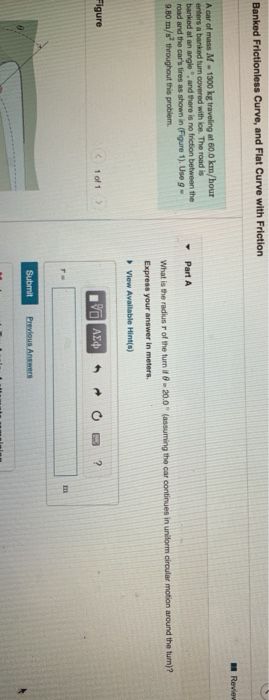Banked Frictionless Curve, and Flat Curve with Friction Reviev A car of mass M - 1300 kg traveling at 60.0 km/hour enfers a banked turn covered with ice. The road is banked at an angle and there is no friction between the road and the car's tires as shown in Figure 1). Use 9 - 9.B0 m/s throughout this problem. Part A What is the radius of the turn it 8 - 20.0 (assuming the car continues in uniform circular...